#Function Repository Resource:

# VennDiagram

Generate the Venn diagram associated to a logical expression or collection of sets

Contributed by: Jack Heimrath
 ResourceFunction["VennDiagram"][expr] generates the Venn diagram associated to expr. ResourceFunction["VennDiagram"][{s1,s2,…}] generates the Venn diagram associated to the sets s1,s2,….

## Details

The input must be a valid logical expression containing between two and five variables or a list of between two and five sets.
The following Options can be used:
 "DiagramLegends" "SwatchLegend" specifies which legends to use "EquationForm" "AND" converts the legend expressions to the specified form 185 the absolute size at which to render the graphic
The "DiagramLegends" and "EquationForm" Options are only applicable to a Venn diagram of a list of lists.
The following settings for "DiagramLegends" can be given:
 "SwatchLegend" generates a legend that associates colored swatches to appropriately colored regions of the diagram "MouseOver" highlights the region under the cursor and displays the equation associated to it
The following settings for "EquationForm" can be given:
 no equation "DNF","SOP" disjunctive normal form, sum of products "CNF","POS" conjunctive normal form, product of sums "ESOP" exclusive sum of products "ANF" algebraic normal form "NOR" two-level Nor and Not "NAND" two-level Nand and Not "AND" two-level And and Not "OR" two-level Or and Not "IMPLIES" Implies and Not "ITE","IF" If and constants "BFF" BooleanFunction form "BDT" Boolean decision tree

## Examples

### Basic Examples (3)

Generate a Venn diagram representing a logical expression:

 In:=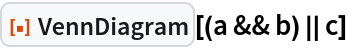Out=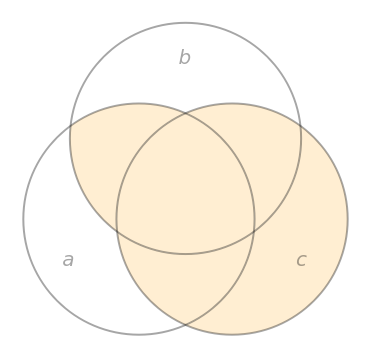In:=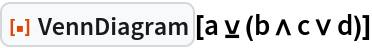Out=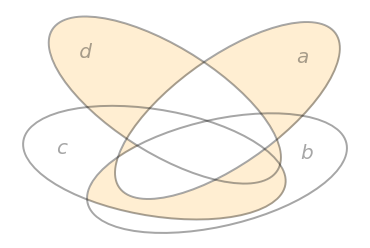In:=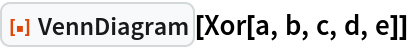Out=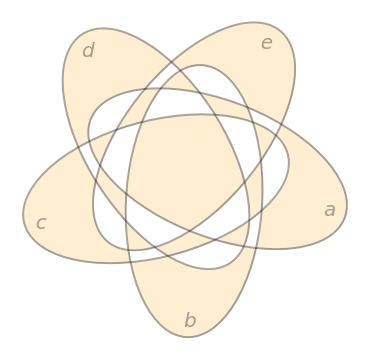VennDiagram accepts Boolean functions as input:

 In:=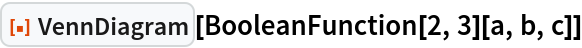Out=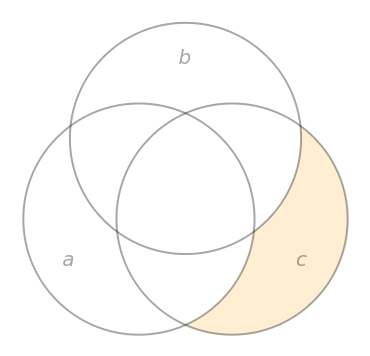Generate a Venn diagram for explicitly defined sets:

 In:=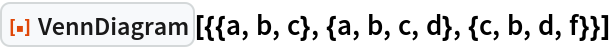Out=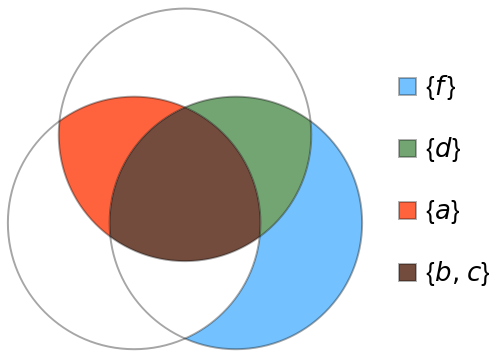### Options (3)

#### EquationForm (1)

Change the canonical normal form of equations used to describe particular sets:

 In:=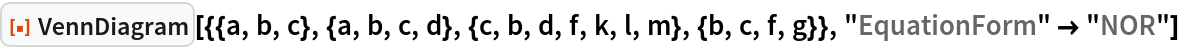Out=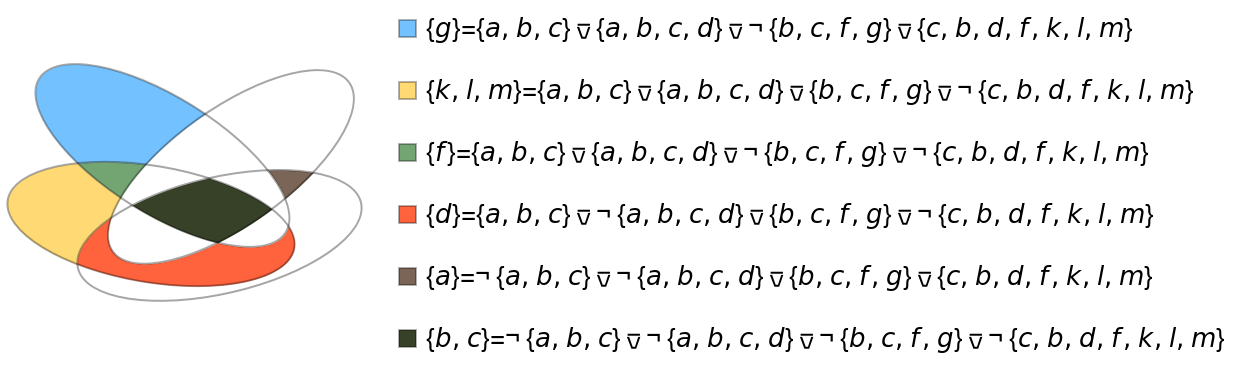#### MouseOver (1)

Only display the content and equation of the region beneath the cursor:

 In:=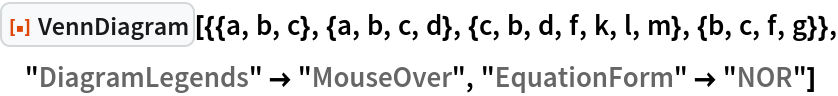Out=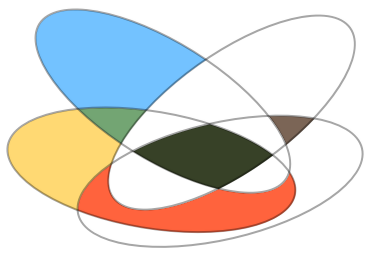#### ImageSize (1)

Create Venn diagrams of various sizes:

 In:=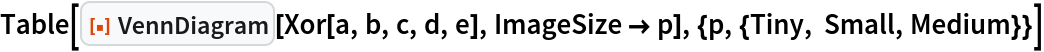Out=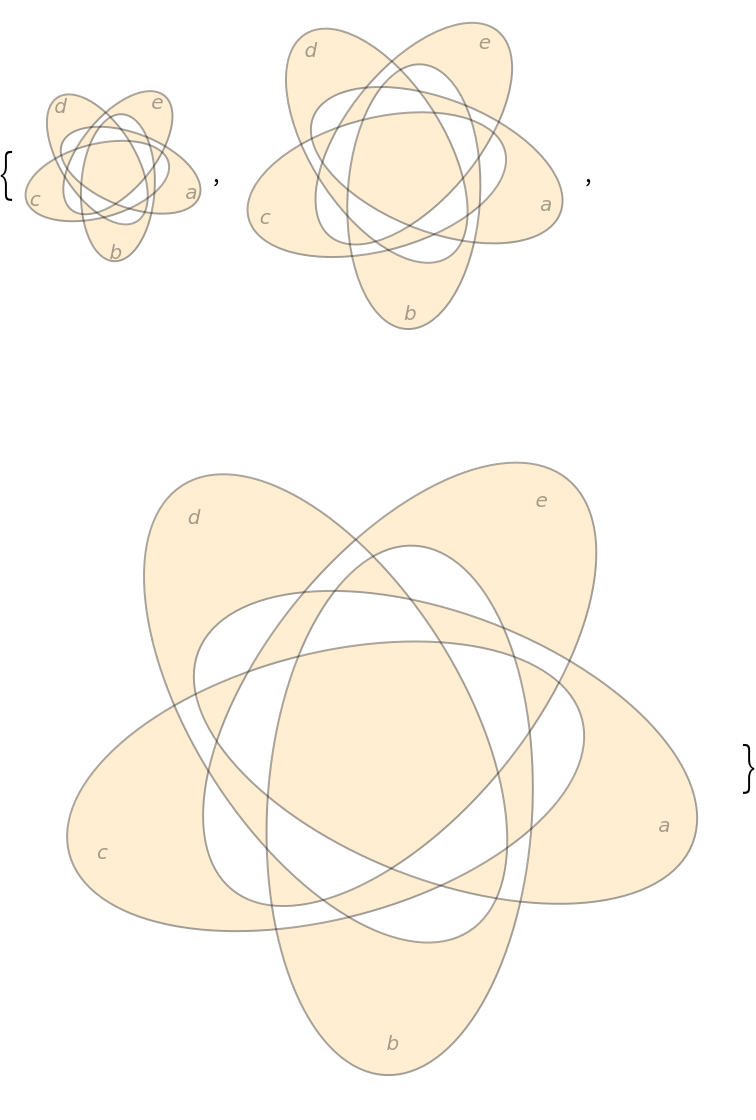### Possible Issues (1)

VennDiagram requires at least 2, but no more than 5 variables or sets:

 In:=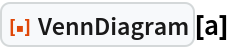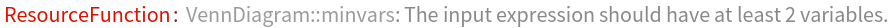Out=In:=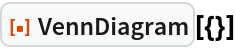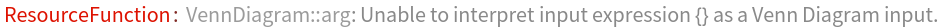Out=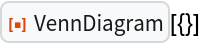## Author Notes

Currently only works for expressions with 2 to 5 variables or for a list of 2 to 5 sets.